The WINKS Professional Edition includes all of the features of the WINKS BASIC EDITION plus... Grubbs test for outliers & Tukey's outlier test Advanced Tabulation allows breakdown of tables by three factors with various statistics included in each cell. The Mantel-Haenszel procedure allows analysis of multiway tables (useful in meta-analysis, when you want to pool information from several 2x2 tables or for the analysis of two dichotomous variables while adjusting for third.) Inter/Rater Reliability & Kappa allows analysis of reliability data that includes both Kappa and Weight Kappa analyses including confidence intervals Two Factor Analysis of Variance can handle unbalanced data and includes graphs and post hoc multiple comparisons Two-way ANOVA with Repeated Measures includes graphs and post hoc tests. Analysis of Covariance (ANCOVA) includes graphs and post hoc tests Three-Way ANOVA Polynomial Regression up to 6th order sextic regression with graphs Stepwise regression for multiple linear regression modeling All possible subsets regression finds best models for multiple linear regression Simple Logistic Regression for simple logistic modeling with a binary or continuous independent variable, including calculating predictions from model and includes odds ratio with 95% confidence intervals Multiple Logistic Regression -- allows you to fit up to 10 independent variables in a logistic model, includes calculation of parameters and odds rations with 95% confidence intervals. Bland-Altman plots for comparison of two similar measures, includes a plot of equality as well as the Bland-Altman plot Multiple comparisons (Newman-Keuls, Tukey HSD, or Scheffé), calculate custom post hoc multiple comparisons that include a graphical summary of results Time series analysis including data plotting, autocorrelations, partial autocorrelations, estimating parameters, differencing and forecasting. The program allows you to estimate the parameters of an ARMA model and create a forecast using your model (using three forecasting techniques). The forecast can then be displayed and captured as numbers in a file. Quality Control charts and procedures allows you to create X-Bar Charts, R-Charts, S-Charts, EWMA, MR-Charts, I-Charts and P-Charts. It will automatically calculate 3-sigma limits, or you can specify your own limits. Data replicates can be balanced or unbalanced. X-Bar Charts can be displayed on the same screen with an R-Chart or an S-Chart. The Pareto Chart procedure allows you to display Pareto charts, which are used to determine priorities for quality improvement. The by-group feature allows you to display charts by a grouping factor (i.e., day of week, operator.) Following are sample graphs from WINKS Professional SDA: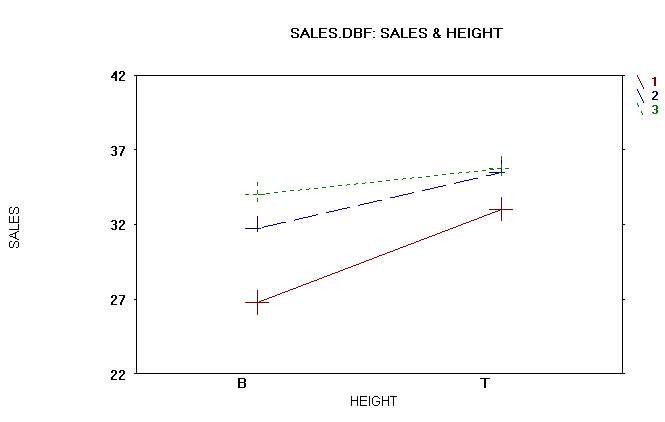(Interaction plot from Two-Way ANOVA)Example ANCOVA Plot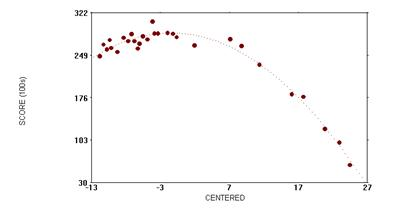Polynomial regression plot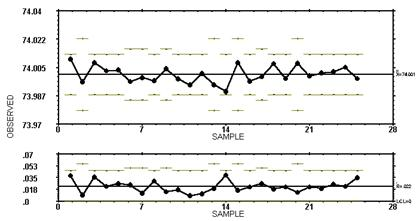Quality Control Plot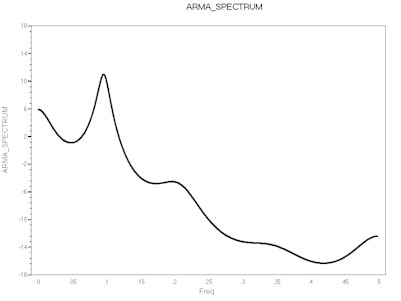Time series spectrum plotROC Curve Logistic Regression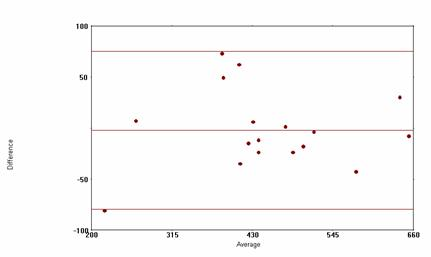Bland Altman Plot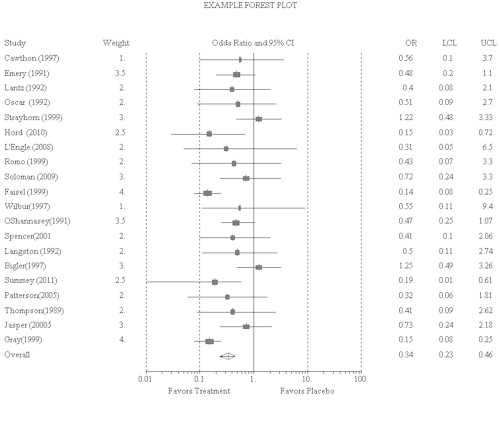Forest Plot (Meta-Analysis) Some procedures in WINKS SDA Professional require Windows XP or higher.